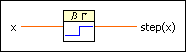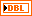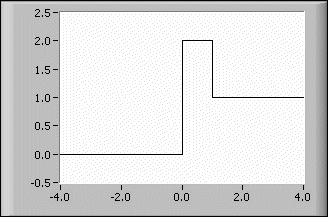# Step Function VI

## LabVIEW 2018 Help

Edition Date: March 2018
Part Number: 371361R-01
View Product InfoLabVIEW 2016 HelpLabVIEW 2017 HelpLabVIEW 2018 HelpLabVIEW 2019 HelpLabVIEW 2020 Help

Owning Palette: Gating Functions VIs

Requires: Full Development System

Generates the step function for any real number x.x is any real number.step(x) is the value of step(x) for the given value of x.

## Step Function Details

The following equation defines the step function.

step(x) = 0 if x < 0 and 1 elsewhereNote  You can define more complex functions by varying and combining the Step Function, Spike Function, and Square Function VIs, respectively.

The following illustration shows the graph for step(x) + spike(x) in the interval (–4.0, 4.0)